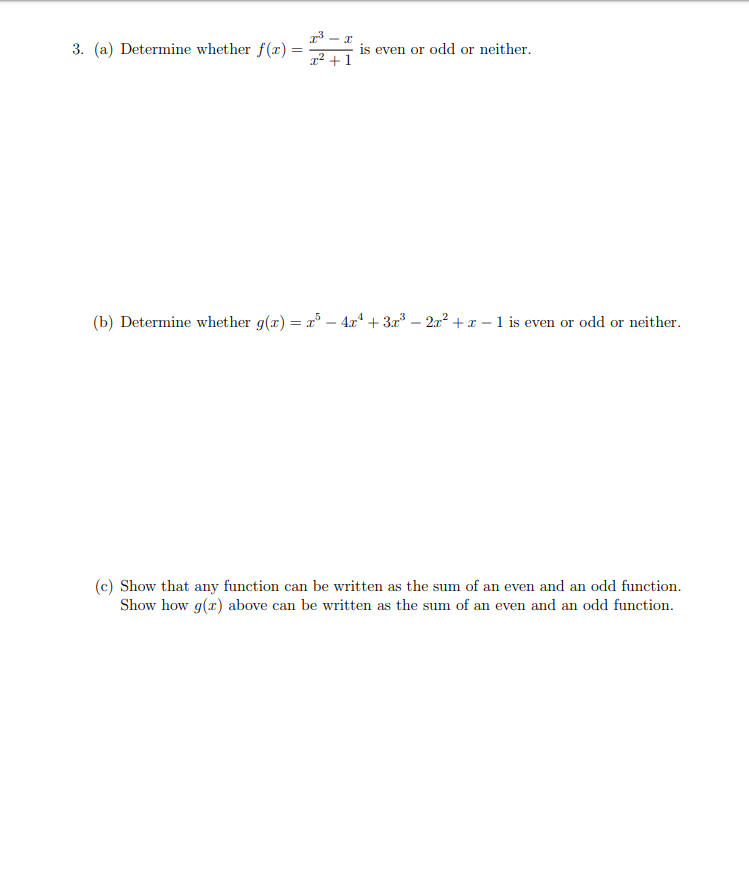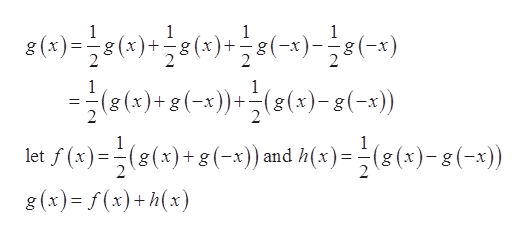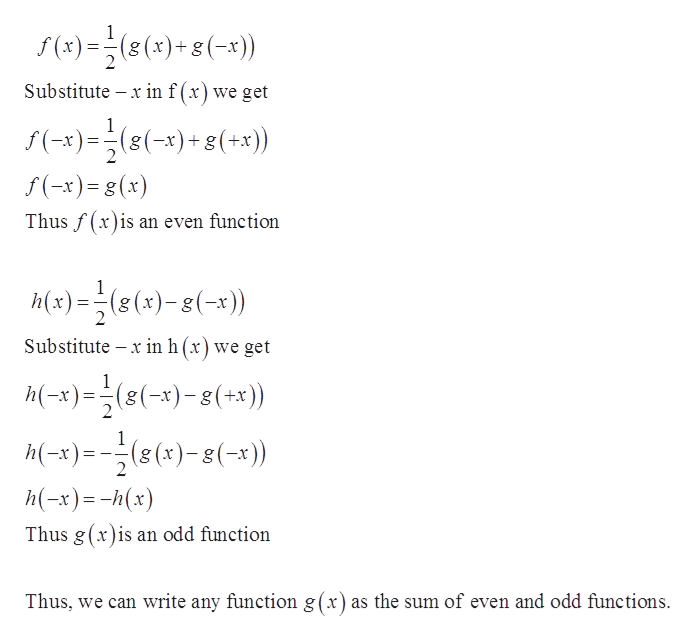# 3. (a) Determine whether f(x)is even or odd or neither(b) Determine whether g(x) = 5 - 4z4 +323 - 2x2 + x _ 1 is even or odd or neither(c) Show that any function can be written as the sum of an even and an odd functionShow how g(z) above can be written as the sum of an even and an odd function

Question
12 views

Practice Question 3.3help_outlineImage Transcriptionclose3. (a) Determine whether f(x) is even or odd or neither (b) Determine whether g(x) = 5 - 4z4 +323 - 2x2 + x _ 1 is even or odd or neither (c) Show that any function can be written as the sum of an even and an odd function Show how g(z) above can be written as the sum of an even and an odd function fullscreen
check_circle

Step 1

Let g(x) be any function we can write it as shown belowhelp_outlineImage Transcriptionclose1 8 (x)())(-x)(-) 1 1 (2 (x)+(-x)(2(x)-g(-x) t()()(-) ) md k()=(()-2(-3) 8 (x) f(x)+h(x) 1 (g(x)+g(-x))and h(x)=(g(x)-(-x) fullscreen
Step 2

Here f(x) and h(x) are even and o...help_outlineImage Transcriptioncloses(x)(()+g(-x)) Substitute x in f (x ) we get f(-x)=(8(-x)8(+x)) gg f(-x)=(x) Thus f(x)is an even function Mx)(s(x)-(- )) Substitute x in h (x) we get h(-x)(x)-(+x)) M(-) )-g(-)) h(-x)h(x) Thus g(x)is an odd function Thus, we can write any function g(x) as the sum of even and odd functions fullscreen

### Want to see the full answer?

See Solution

#### Want to see this answer and more?

Solutions are written by subject experts who are available 24/7. Questions are typically answered within 1 hour.*

See Solution
*Response times may vary by subject and question.
Tagged in

### Other